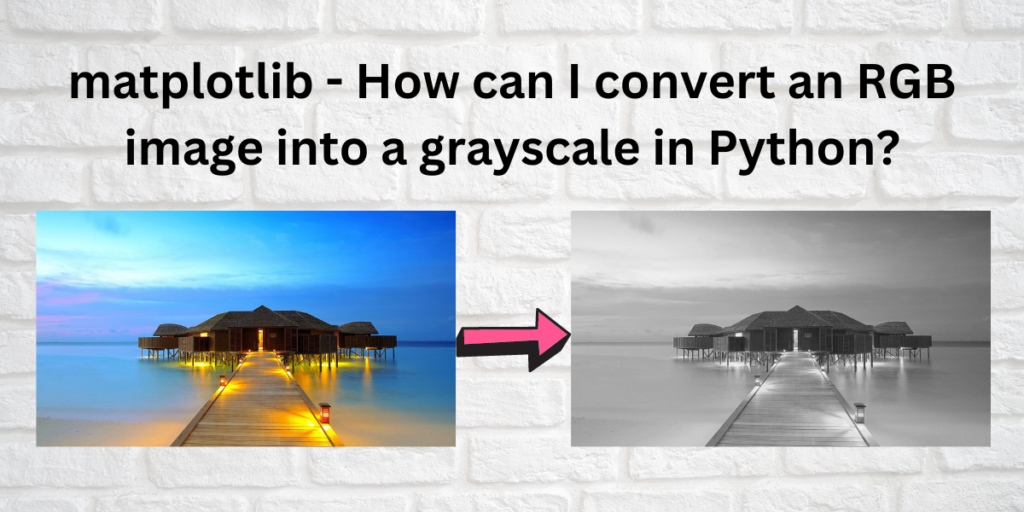# Convert an RGB image into grayscale using MatplotlibThe process of turning a colored image into black and white is known as gray scaling. A colorful image can be converted to monochrome in python using more than one method. In this article, we will look at one of the many ways for doing the same.

The four main methods in which gray scaling can be done in python are:

• Using Matplotlib and numpy libraries
• Using CV2.cvtcolor() function
• Using the cv2.read() function where flag=zero
• Using scikit learn and matplotlib.

For now, we will discuss the methods involving matplotlib. But before we do that, we will look at the advantages of using a monochrome image in image processing instead of an RGB image.

## Why use black and white images?

• Using a monochrome image helps in reducing the complexity of computations which involve image processing.
• Enhances easy visualization as it converts an image having three dimensions (R, G, and B) to a 2 dimensional one highlighting the shadows and the bright portions of a picture.
• It makes image recognition easier.
• Converting a RGB image to monochrome compresses an image to it’s bare minimum size which is useful.
• It helps in improving the speed of a program by cutting it’s processing time by a factor of three or maybe sometimes even four.
• It converts signal to noise as in many programs complex colors do not help in identifying any special features.
• To reduce the pixel data which might take up additional and unnecessary space during processing.

## Why Matplotlib?

Matplotlib is a python library that makes data visualization in python easier and more easily digestible. It provides a wide range of functions for image editing and manipulation. Coupled with NumPy or scikit modules, the matplotlib library can be a powerful tool for image processing purposes.

Let’s convert an RGB image to grayscale using matplotlib. We will use numpy and matplotlib and then the scikit library along with matplotlib.

Also read: Data Visualization using matplotlib.pyplot.scatter in Python.

## Prerequisites for Getting Started

You would need to install some required modules and libraries for your system before carrying out any image processing operations. Run the following code in your command prompt in administrator mode for proper installation:

```pip install numpy
pip install matplotlib
pip install scikit-learn
pip install scikit-image
```

Note: If you’re using windows, you might get an import error (DLL file not found) while importing some of the matplotlib functions. To avoid this, you can download the DLL files from here, msvcp71.dll and/or msvcr71.dll and save them to your `C:\Windows\System32` or` C:\Windows\SysWOW64` folder. Or simply, download Visual C++ Redistributable for Visual Studio 2015 (this is usually more easy and effective).

To know about more such python modules, read Introduction to Python Modules.

### Method 1: Using matplotlib and Numpy

Numpy or the numerical python library supports a large number of high level mathematical functions involving N dimensional arrays and matrices. Now, we will look at how numpy along with matplotlib can be used to turn a colored image into a neutral one.

In this tutorial, we will use the following image called “seaside.jpg”. You can use whatever image you see fit. If you want you can download this image from here.

We will use the numpy.dot() function and create a function to convert the above image into a grayscale image.

```#importing required modules
import numpy as np
import matplotlib.pyplot as plt
import matplotlib.image as mpimg

#function using np.dot()
def rgb2gray(rgb):
return np.dot(rgb[...,:3], [0.299, 0.587, 0.144])
plt.imshow(img_rgb)
#display the original image
plt.show()

#converting the image to monochrome
img_gray = rgb2gray(img_rgb)
plt.imshow(img_gray, cmap=plt.get_cmap('gray'))
plt.savefig('seasidegray.jpg')
#display the black and white image
plt.show()
```

Now when you run the above code, you will get two outputs: The first one being the original image, and the second one being the processed image.

### Method 2: Using Matplotlib and Scikit Learn

Scikit learn is a free software that is used for machine learning algorithms in python. It supports numerous operations such as regression, clustering, etc.

Image processing can also be performed using scikit learn. Let’s look at the same.

```#importing required modules and libraries
import matplotlib.pyplot as plt
from skimage import io
from skimage import data
from skimage.color import rgb2gray
from skimage import data
#converting the image into grayscale
gray_img  = rgb2gray(rgb_img)
fig, axes = plt.subplots(1, 2, figsize=(8, 4))
ax = axes.ravel()
#setting the axes of the image
ax.imshow(rgb_img)
ax.set_title("Original image")
ax.imshow(gray_img, cmap=plt.cm.gray)
ax.set_title("Processed image")
#display the processed image
fig.tight_layout()
plt.show()
```

the output would look something like this: